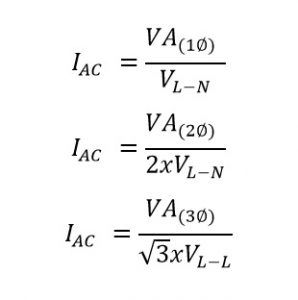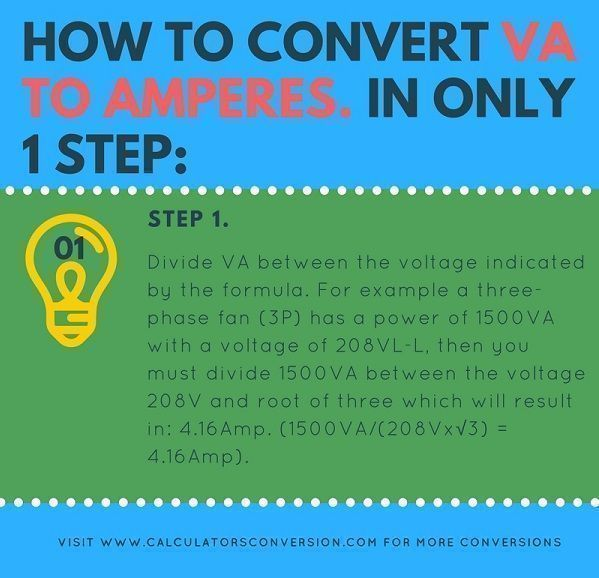# Volt Amp. (VA) to Amp. (Amperes) – Convert, conversion, table and formula

With this calculator you can convert from Volt Amp. (VA) to Amp. (Amperes) automatically, easily, quickly and free any electric power.

We also show how to convert VA to Amps to 1 step, the formula that is used for the conversion and a chart with the main conversions from VA to Amps.

## VA to Amps calculation formula for generator and transformer:• IAC=Ampere.
• VL-L=Volts Line-Line.
• VL-N=Volts Line-Neutral.
• VA=Volt-Ampere.

## How to convert VA to Amp. in only 1 step:Step 1:

Divide VA between the voltage indicated by the formula. For example a three-phase fan (3P) has a power of 1500VA with a voltage of 208VL-L, then you must divide 1500VA between the voltage 208V and root of three which will result in: 4.16Amp. (1500VA/(208Vx√3) = 4.16Amp).

## Definition Amperes and S (VA):

VA: A Volt-ampere, commonly referred to as a VA, is commonly used as a unit of power in obtaining the electrical capacity of circuit breakers, uninterrupted power supplies and wirings.

VA is larger than Watts because loads are inductive such as motors, discharge lighting, reactors and more current is required to keep the magnetic field energized than is -turned into heat (W).

Inductive devices or loads such,. as tansformers and motors having power factor less than 1.0 are generally rated in VA.

Ampere: Amperage is a term often used by electricians, and means electrical current, measured in amperes, or amps. The ampere is the SI unit for electrical current, or the amount of electrical charge that flows through a conductor in a given time. One ampere is a charge of one coulomb — about 6.241 X 1018 electrons — per second flowing past a given point.

## VA to Amps conversion chart:

 VA Phase Volt Amp. 1VA 3 Phase 208Volt 0,0027Amp. 2VA 3 Phase 208Volt 0,0055Amp. 3VA 3 Phase 208Volt 0,0083Amp. 4VA 3 Phase 208Volt 0,011Amp. 5VA 3 Phase 208Volt 0,013Amp. 6VA 3 Phase 208Volt 0,016Amp. 7VA 3 Phase 208Volt 0,019Amp. 8VA 3 Phase 208Volt 0,022Amp. 9VA 3 Phase 208Volt 0,024Amp. 10VA 3 Phase 220Volt 0,026Amp. 20VA 3 Phase 220Volt 0,052Amp. 30VA 3 Phase 220Volt 0,078Amp. 40VA 3 Phase 220Volt 0,104Amp. 50VA 3 Phase 220Volt 0,131Amp. 60VA 3 Phase 220Volt 0,157Amp. 70VA 3 Phase 220Volt 0,183Amp. 80VA 3 Phase 220Volt 0,209Amp. 90VA 3 Phase 220Volt 0,236Amp. 100VA 3 Phase 440Volt 0,13121Amp. 200VA 3 Phase 440Volt 0,262Amp. 300VA 3 Phase 440Volt 0,393Amp. 400VA 3 Phase 440Volt 0,524Amp. 500VA 3 Phase 440Volt 0,656Amp. 600VA 3 Phase 440Volt 0,787Amp. 700VA 3 Phase 440Volt 0,918Amp. 800VA 3 Phase 440Volt 1,049Amp. 900VA 3 Phase 440Volt 1,18Amp. 1000VA 3 Phase 460Volt 1,255Amp. 2000VA 3 Phase 460Volt 2,51Amp. 3000VA 3 Phase 460Volt 3,76Amp. 4000VA 3 Phase 460Volt 5,02Amp. 5000VA 3 Phase 460Volt 6,275Amp. 6000VA 3 Phase 460Volt 7,53Amp. 7000VA 3 Phase 460Volt 8,785Amp. 8000VA 3 Phase 460Volt 10,04Amp. 9000VA 3 Phase 480Volt 10,82Amp. 10000VA 3 Phase 480Volt 12,028Amp. 20000VA 3 Phase 480Volt 24,056Amp. 30000VA 3 Phase 480Volt 36,08Amp. 40000VA 3 Phase 480Volt 48,11Amp. 50000VA 3 Phase 480Volt 60,14Amp. 60000VA 3 Phase 480Volt 72,168Amp. 70000VA 3 Phase 480Volt 84,196Amp. 80000VA 3 Phase 480Volt 96,22Amp. 90000VA 3 Phase 480Volt 108,25Amp.

Rate this calculator VA to Amps:  [kkstarratings]